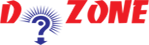﻿ Python Program to Check if a Number is Odd or Even## Python Program to Check if a Number is Odd or Even

Let's learn how to check either a number entered into the program is Odd or Even through python coding.

As we all know that, the number is even if it is divisible by 2. here we use the remainder operator % and if the remainder is not zero then the number is odd.

``````
Input
# Python program to check if the input number is odd or even.
# A number is even if division by 2 give a remainder of 0.
# If remainder is 1, it is odd number.

num = int(input("Enter a number: "))
if (num % 2) == 0:
print("{0} is Even".format(num))
else:
print("{0} is Odd".format(num))
```
```
``````
Output I
Enter a number: 61
61 is Odd
```
```
``````
Output II
Enter a number: 20
20 is Even
```
```

## Other Python Programs

PythonTraining, 258-FF , Katewa Nagar, New Sanganer Rd, Jaipur, 302019